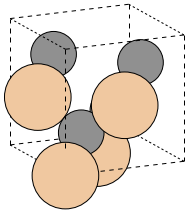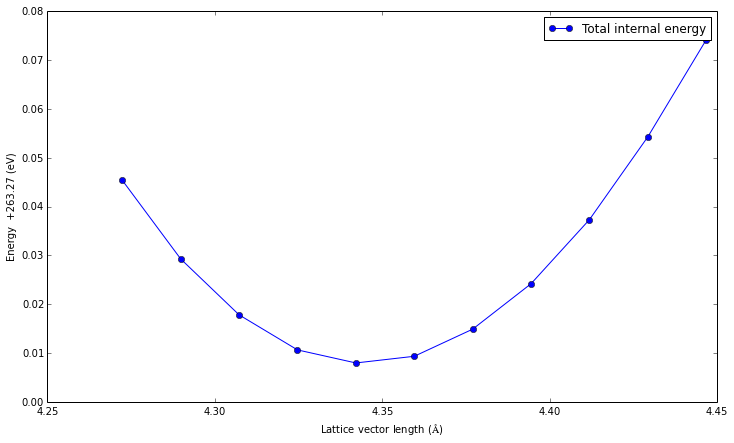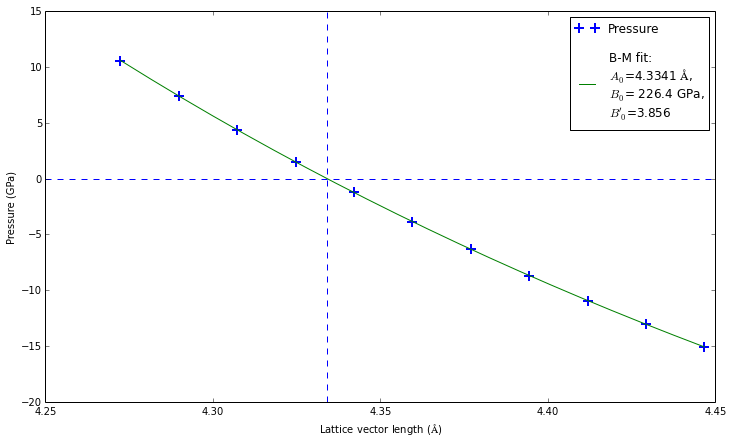# Setup of crystal structure, energy and stress calculations¶

This example uses a local installation of Quantum Espresso. Without such installation you will not be able to execute this tutorial. However, you can still read it and lern from it. The "Remote execution" tutorial contains similar material and is configured for remote execution of Quantum Espresso.

To use the qeutil system you need to do three things first.

• Read a basic introduction to the iPython interpreter and the notebook environment (The links to the documentation are at the top of the page under menu "Help"). Note particularly "Keyboard shortcuts" document.
• Import a set of libraries into your notebook environment (see below).
• Configure the qeutil for your particular computing setup

The qeutil library is built as an extension to the ASE library. Many of the presented functions depend on the functionality of the ASE library. Thus, it is highly recommended to familiarize yourself with its structure and function. The full documentation is available on the web.

During working out the examples pay attention to the comments in the code, which start with the "#" character and continue to the end of the line. They are added for your convenience - to provide detailed descriptions of the procedure.

The whole notebook system is written in the python language. You do not need high-level knowledge of the languade to understand the tutorials. However, some familiarity with its syntax is still required. Introduction to the python language may be found in the Help menu, if you need it. Please note that the indentation in the python code is important. The blocks of code are defined by the common indentation.

## Configuring¶

This part is a little bit more complicated. This notebook is configured for a local execution of the Quantum Espresso programs. The qe-util has some support for the remote execution with a help of the queue management system. This topic is covered by the second notebook named "Remote calculation".

For this exercise just review the configuration in the second cell and verify that the commands actually execute the Quantum-Espresso programs.

The following notebook includes a minimal set of libraries required for it to run (see the first cell of this notebook). You may need additional libraries if you extend the analysis presented below. It is as easy as adding additional 'import' clauses at the beginning.

In :
# Import the basic libraries

# ASE system
import ase
from ase import Atom, Atoms
from ase import io
from ase.lattice.spacegroup import crystal

# Spacegroup/symmetry library
from pyspglib import spglib

# The qe-util package
from qeutil import QuantumEspresso

# iPython utility function
from IPython.core.display import Image


## Configuration¶

Configure qe-util library for local execution of the Quantum Espresso on four processors. The commands should execute the following programs from the Quantum Espresso package:

• pw.x the basic program for calculating electronic structure and basic static properties of the crystal such as:
• Electronic structure
• Energy
• Stress tensor
• Charge distribution
• ph.x the program for calculating the a second derivative of the energy with respect to atomic displacements or unit cell deformations.
• matdyn.x the program for processing dynamical matrix of the crystal.
• q2r.x the program for the transformation of the dynamical matrix into a matrix of force constants in real space.

The commands should execute the pw.x program of the Quantum Espresso suite using pw.in and pw.out files as input and output respectively. The example below is a fairly standard command for running the pw.x on the four-core PC.

In :
# Configure qe-util for local execution of the Quantum Espresso on four processors
QuantumEspresso.pw_cmd='mpiexec -n 4 pw.x < pw.in > pw.out'


## Crystal definition¶

Here we define a $\beta$-SiC crystal: a cubic zincblende crystal with a spacegroup F-43m (space group number 216) and an experimental lattice constant (here, A=4.3596 A). The atomic positions are specified in the fractional (crystallographic) coordinates; i.e. coordinates measured in units of lattice constants in the coordinate system defined by lattice vectors. The unit cell is specified as three lengths (in angstrom) of the lattice vectors and three angles (in degrees) between these vectors. The crystal axes are oriented in the conventional way in the Cartesian (X,Y,Z) coordinate system:

• Vector $\vec{A}$ is along the X axis
• Vector $\vec{B}$ is in the XY plane
In :
a=4.3596                                         # Lattice constant in Angstrom
cryst = crystal(['Si', 'C'],                     # Atoms in the crystal
[(0, 0, 0), (0.25, 0.25, 0.25)], # Atomic positions (fractional coordinates)
spacegroup=216,                  # International number of the spacegroup of the crystal
cellpar=[a, a, a, 90, 90, 90])   # Unit cell (a, b, c, alpha, beta, gamma) in Angstrom, Degrees


We can display the picture of the crystal. The ase.io.write procedure, used here, is a very flexible tool. It can write the crystal in numerous formats, it can even run external tools for rendering a 3D scene. Here, we just use a simple renderer build into the write procedure to store a picture of the crystal into a disk file and then to display it.

In :
# Write the image to disk file
ase.io.write('crystal.png',       # The file where the picture get stored
cryst,               # The object holding the crystal definition
format='png',        # Format of the file
show_unit_cell=2,    # Draw the unit cell boundaries
rotation='115y,15x', # Rotate the scene by 115deg around Y axis and 15deg around X axis
scale=30)            # Scale of the picture

# Display the image
Image(filename='crystal.png')

Out:Check the spacegroup (symmetry) of our creation. The spglib library provides various functions dealing with the symmetry of crystals. For example, it has a symmetry finder, which can identify the symmetry group of the crystal. Here, we use this function to check if the structure we have created is indeed a zinc-blende cubic crystal (F-43m group).

In :
print 'Space group:', spglib.get_spacegroup(cryst)

Space group: F-43m      (216)


## Defining the Calculator¶

Now, when we have our crystal build we need to define a Calculator for our computations. It is done by creation of QuantumEspresso object, specifying various parameters for the calculation. The same calculator may be used for a number of structures and a number of computations. It is a provider of functions which allow the crystal object to respond to questions such as: "What is your total energy?". You need to specify a number of parameters for the calculator which are specific to the case. The parameters used here should not be used in the production runs. They are defined just for the presentation purposes. For a real calculation you need to select the parameters according to the case you are dealing with. Unfortunately there is no simple "rule of thumb" for these parameters. The list of possible parameters covers a fairly complete set of parameters used by Quantum Espresso package. For the description of possible parameters you need to consult the documentation on the Quantum Espresso website, particularly the document describing input parameters

The meaning of the parameters in greater detail:

• label -- This is a label for the calculator. It is used as a first part of the directory name used by the calculator to store and execute the calculations.
• kpts -- A list of k-vectors for the sampling of the Brillouin zone. Here it is specified as a grid size (n x m x k) which is a typical approach. It may be also specified as a list of k-vectors
• xc, pp_type, pp_format -- These are the parts of the name of the used pseudopotentials. The pseudopotential name is constructed as:

(Element_symbol)(xc)(pp_type).(pp_format)

• ecutwfc -- Cut-off energy (in Ry) for the plane waves used in the calculation. This needs to be adjusted according to the pseudopotential used.
• use_symmetry -- Controls use of symmetry in the calculation. If set to True the calculator will internally extract a primitive unit cell out of the crystal and perform all calculations on the primitive unit cell.
In :
# Create a Quantum Espresso calculator for our work.
# This object encapsulates all parameters of the calculation,
# not the system we are investigating.
qe=QuantumEspresso(label='SiC',                    # Label for calculations
wdir='calc',                # Working directory
pseudo_dir='../../pspot',   # Directory with pseudopotentials
kpts=[8,8,8],               # K-space sampling for the SCF calculation
xc='pz',                    # Exchange functional type in the name of the pseudopotentials
pp_type='vbc',              # Variant of the pseudopotential
pp_format='UPF',            # Format of the pseudopotential files
ecutwfc=70,                 # Energy cut-off (in Rydberg)
use_symmetry=True)          # Use symmetry in the calculation ?

# Check where the calculation files will reside.
print qe.directory

calc/SiC.mjVUKG

In :
# Assign the calculator to our system
cryst.set_calculator(qe)


## Computing - single-point calculation¶

We are ready to perform our first Quantum Espresso calculation. It is as simple as asking the crystal for its energy or stress tensor.

You just need to call an appropriate function: e.g. cryst.get_stress()

The calculation may take some time (5-30s, depending on your system). Be patient.

Note 1: The default notation for stress tensors used here is a Voigt notation. Where the independent components of a symmetric stress tensor $\sigma$ are collected into a 6-component quantity (note: it is not a vector in the tensor analysis sense). This is a common notation in the tensor algebra of symmetric tensors. The components of the stress tensor in the Voigt notation are: $$[\sigma_{xx}, \sigma_{yy}, \sigma_{zz}, \sigma_{yz}, \sigma_{xz}, \sigma_{yz}]$$

Note 2: The convention for signs of external pressure is opposite to the sign of stress.

Note 3: In case of the interrupted calculation it is easy to recover from such a crash by adding recover = .true. to the input file.

In :
# Run the calculation to get stress tensor (Voigt notation, in eV/A^3) and pressure (in kBar)
print "Stress tensor   (Voigt notation eV/A^3):", cryst.get_stress()
print "External pressure                (kBar):", cryst.get_isotropic_pressure(cryst.get_stress())*1e-3

Stress tensor   (Voigt notation eV/A^3): [ 0.02404228  0.02404228  0.02404228 -0.          0.          0.        ]
External pressure                (kBar): -38.52

In :
# Do the same but get the results in GPa
# Note that this time we get the results immediately.
# We did not change the system, so it is not necessary to repeat the calculation.
print "Stress tensor  (Voigt notation, GPa):", cryst.get_stress()/ase.units.GPa
print
print "Stress tensor (Tensor notation, GPa):"
print cryst.get_stress(voigt=False)/ase.units.GPa
print
print "External pressure              (GPa):", cryst.get_isotropic_pressure(cryst.get_stress())*1e-4

Stress tensor  (Voigt notation, GPa): [ 3.852  3.852  3.852 -0.     0.     0.   ]

Stress tensor (Tensor notation, GPa):
[[ 3.852  0.     0.   ]
[ 0.     3.852 -0.   ]
[ 0.    -0.     3.852]]

External pressure              (GPa): -3.852


## Computing - a series of calculations¶

One of the advantages of this system is that you can run a series of calculations automatically, as is illustrated below. For example we can find a minimum of energy of our crystal - which is its equilibrium lattice constant. To do this it is needed to modify the crystal at each turn of the loop and collect the results. Alternatively you can create a whole bunch of systems and calculators and run them all at once. If you have many CPUs this may considerably speed up the calculation. Here we will do a sequential run. The topic of parallel execution will be covered in the "Remote calculation" notebook.

In :
# A sequential run for a series of lattice constants

# We will store the results in this list
result=[]

# Our prototype crystal is just a copy of the structure defined above
cr=Atoms(cryst)

# It needs a calculator as well. This may be the same calculator or we can define a separate one.
cr.set_calculator(qe)

print " Scale     A(A)     Energy(eV)   Pressure(GPa) "
print "==============================================="

# Iterate over scales between 98% and 102% of the starting unit cell size.
# We use 11 points in the interval
for x in linspace(0.98,1.02,11):
# Modify the crystal by scaling the lattice vectors
cr.set_cell(cryst.get_cell()*x,scale_atoms=True)

# Calculate energy and stress and store the results in the result list
result.append([x, x*cryst.get_cell()[0,0], cr.get_potential_energy(), 1e-4*cr.get_isotropic_pressure(cr.get_stress())])

# Print it as well
print "% 5.03f   % 6.04f    %+6.4f      % +8.3f " % tuple(result[-1])

# Prepare the collected data for plotting
# This will make an array (matrix) out of a list and transpose it for easier access later
# Transposing the matrix means that we can specify just a column to get the whole column as a vector.
result=array(result).T

# Let us save our calculated data to a file.
# To have data in columns we need to transpose the array again.
# This is a consequence of the row-column conventions and has no deeper meaning.
savetxt('e+p-vs-a.dat',result.T)

 Scale     A(A)     Energy(eV)   Pressure(GPa)
===============================================
0.980    4.2724    -263.2246       +10.575
0.984    4.2898    -263.2407        +7.403
0.988    4.3073    -263.2522        +4.380
0.992    4.3247    -263.2594        +1.501
0.996    4.3422    -263.2620        -1.242
1.000    4.3596    -263.2607        -3.852
1.004    4.3770    -263.2551        -6.335
1.008    4.3945    -263.2458        -8.694
1.012    4.4119    -263.2327       -10.933
1.016    4.4294    -263.2157       -13.058
1.020    4.4468    -263.1959       -15.062


## Analysis¶

All plotting in the notebook environment is done by the matplotlib graphics library. It is a very flexible and useful tool for scientific plotting. You will find an extensive documentation on the web. It is linked in the "Help" menu above. Below we will plot the energy and stress curves and find the lattice constant corresponding to the zero stress (minimum energy).

In :
# Let us plot the results and save the figure
figsize(12,7)

# To make the plot nicer we define a shift to energy.
# Rounding to second decimal digit in eV
E0=round(min(result)-(max(result)-min(result))/20,2)

# Plot the result
plot(result,     # Arguments (x-axis)
result-E0,  # Values (y-axis)
'o-',          # Symbol and line style
label='Total internal energy')

# Set the axes labels
xlabel('Lattice vector length ($\AA$)')
ylabel('Energy %+8.2f (eV)' % (-E0))

# Store the figure
savefig('e-vs-a.pdf')## Fitting the Equation of State¶

Below we will fit the Birch-Murnaghan logarithmic equation of state to our lattice constant-pressure data to find:

• Equilibrium lattice constant $A_0$
• Bulk modulus $B_0$
• Derivative of bulk modulus $B'_0$

The Birch-Murnaghan equation of state has a following form:

$$P(V)=\frac{B_0}{B'_0} \left[\left(\frac{V_0}{V}\right)^{B'_0}-1\right]$$

To fit this formula to our data points we use a standard least-squares non-linear optimization procedure leastsq from the optimize module of the SciPy library. The documentation for this library is also included in the "Help" menu.

In :
# Lets do the same with pressure.
# But this time let us fit a Birch-Murnaghan equation of state to the data

# We need a fitting package from scipy
from scipy import optimize

# Define a B-M eos function
def BMEOS(v,v0,b0,b0p):
return (b0/b0p)*(pow(v0/v,b0p) - 1)

# Define functions for fitting
# The B-M EOS is defined as a function of volume.
# Our data is a function of lattice parameter A^3=V
# We need to convert them on-the-fly
fitfunc = lambda p, x: [BMEOS(xv**3,p**3,p,p) for xv in x]
errfunc = lambda p, x, y: fitfunc(p, x) - y

figsize(12,7)
# Plot the data
plot(result,result,'+',markersize=10,markeredgewidth=2,label='Pressure')

# Fit the EOS

# Create a data array: lattice constant vs. isotropic pressure
ap=array([result,result])

# Estimate the initial guess assuming b0p=1
# Limiting arguments
a1=min(ap)
a2=max(ap)
# The pressure is falling with the growing volume
p2=min(ap)
p1=max(ap)

# Estimate the slope
b0=(p1*a1-p2*a2)/(a2-a1)
a0=(a1)*(p1+b0)/b0

# Set the initial guess
p0=[a0,b0,1]

# Fitting
# fit will receive the fitted parameters,
# and value of succ indicates if fitting was successful
fit, succ = optimize.leastsq(errfunc, p0[:], args=(ap,ap))

# Ranges - the ordering in ap is not guaranteed at all!
# In fact it may be purely random.
x=numpy.array([min(ap),max(ap)])
y=numpy.array([min(ap),max(ap)])

# Plot the P(V) curves and points for the crystal

# Mark the center at P=0, A=A0 with dashed lines
axvline(fit,ls='--')
axhline(0,ls='--')

# Plot the fitted B-M EOS through the points,
# and put the fitting results on the figure.
xa=numpy.linspace(x,x[-1],20)
plot(xa,fitfunc(fit,xa),'-',
label="\nB-M fit:\n$A_0$=%6.4f $\AA$,\n$B_0$=%6.1f GPa,\n$B'_0$=%5.3f  " % (fit, fit, fit) )

legend()
xlabel('Lattice vector length ($\AA$)')
ylabel('Pressure (GPa)')

# Save our figure
savefig('p-vs-a.pdf')In :